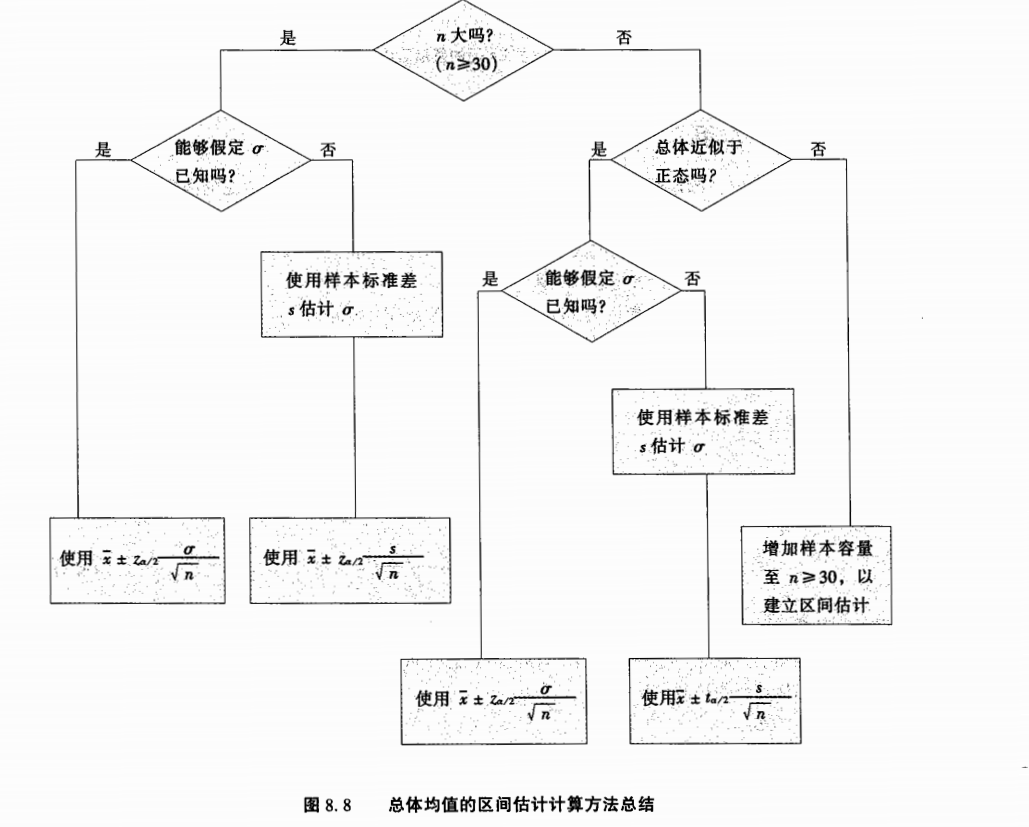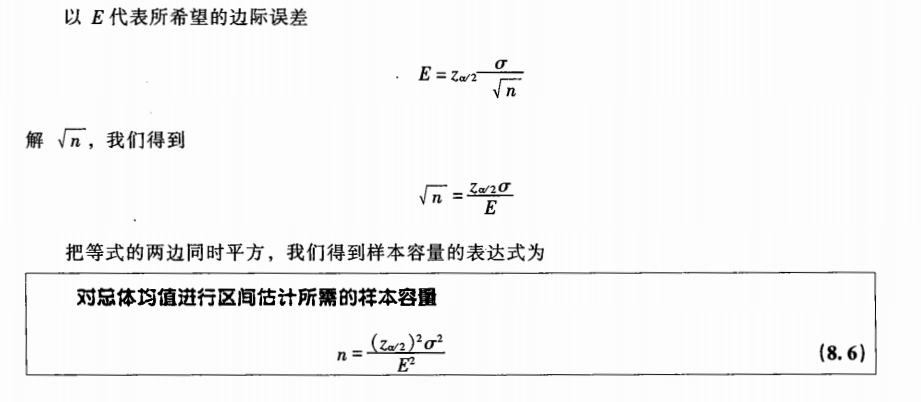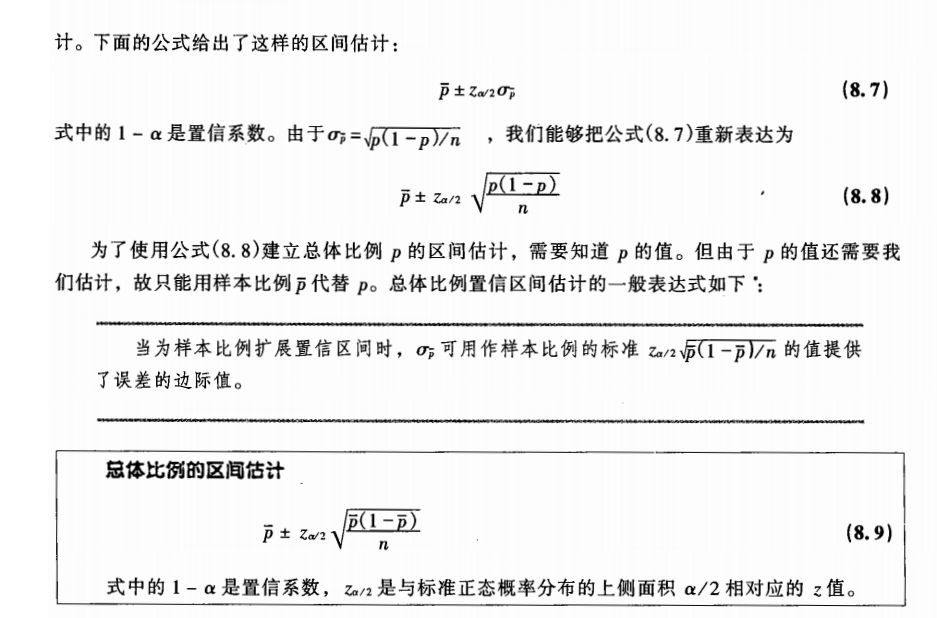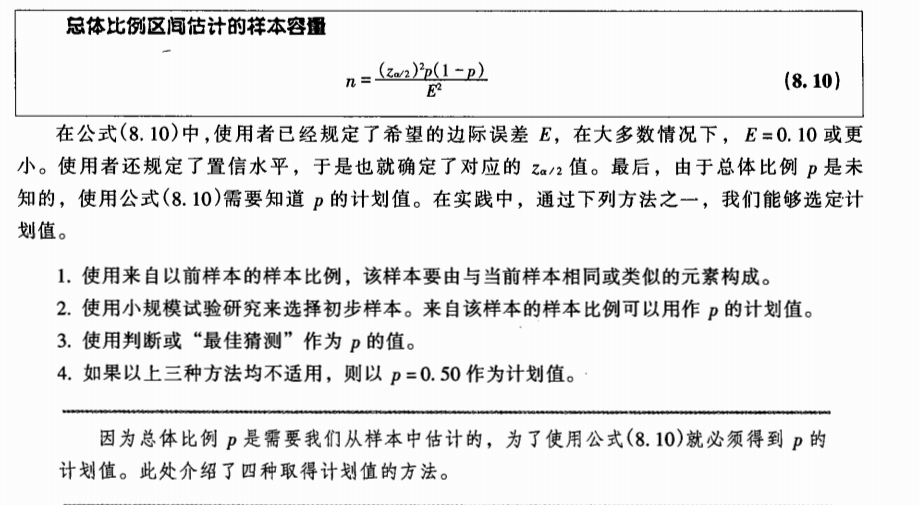• '''统计数据分布''' '''载入数据''' relation=np.loadtxt(path, dtype=float, delimiter=" ") total_list=relation '''求取最大值与最小值的差值''' base=math.ceil(max(total_list))-int(min(total_list)...
def distribution(path):
'''统计数据分布'''
'''载入数据'''
relation=np.loadtxt(path, dtype=float, delimiter=" ")

total_list=relation

'''求取最大值与最小值的差值'''
base=math.ceil(max(total_list))-int(min(total_list))

'''设置分布间隔'''
countblock=10
block=base/countblock

mini=int(min(total_list))
maxi=math.ceil(max(total_list))

intervals = {'%f~%f'%(mini+block*x,mini+block*(x+1)):0 for x in range(countblock)}
for ls in total_list:
for interval in intervals:
start,end = tuple(interval.split('~'))
if float(start)<=ls<=float(end):
intervals[interval] +=1
for i in intervals:
print(i,intervals[i])

print(intervals[i]/len(total_list))

自由组合 生成迭代序列

import itertools
'''自由组合生成迭代序列'''
loop_val = [range(index-5,index),range(index,index+5)]
'''*代表解压'''
ls1 = itertools.product(*loop_val)




展开全文• 通过itertools中的groupby进行统计区间可以自定义，我定义的是10. from itertools import groupby lst=[] with open(path,'r',encoding='utf-8') as f: lines=f.readlines() for i,line in enumerate(lines)...
通过itertools中的groupby进行统计，区间可以自定义，我定义的是10.

from itertools import groupby

lst=[]
with open(path,'r',encoding='utf-8') as f:
lines=f.readlines()
for i,line in enumerate(lines):
cnt=int(line.strip())
lst.append(cnt)
print(len(lst))
for k,g in groupby(sorted(lst),key=lambda x:x//10):
print('{}-{}:{}'.format(k*10,(k+1)*10-1,len(list(g))))


注意：

1、list定义，不能与内置的函数list重名；

2、字典取值用mydit['key']而不是mydict('key')

展开全文• 7.4抽样分布任意一个样本统计量的概率分布被称为该统计量的抽样分布、 x¯\overline x 的数学期望等于从总体中抽取样本的总体均值 E(x¯)=μ E(\overline x) = \mu x¯\overline x 的标准差 有限总体σx¯=N−nN...
7.4抽样分布

任意一个样本统计量的概率分布被称为该统计量的抽样分布、 x¯<!--//--><![CDATA[//><!--
\overline x
//--><!]]> 的数学期望等于从总体中抽取样本的总体均值 E(x¯)=μ<!--//--><![CDATA[//><!--
E(\overline x) = \mu
//--><!]]>x¯<!--//--><![CDATA[//><!--
\overline x
//--><!]]> 的标准差
有限总体σx¯=N−nN−1−−−−−−√(σn√)<!--//--><![CDATA[//><!--
\sigma_\overline x= \sqrt {\frac{N-n}{N-1}}(\frac{\sigma}{\sqrt{n}})
//--><!]]>
无限总体 σx¯=σn√<!--//--><![CDATA[//><!--
\sigma_\overline x=\frac{\sigma}{\sqrt{n}}
//--><!]]>
p抽样分布
期望 E(p¯)=p<!--//--><![CDATA[//><!--
E(\overline p)= p
//--><!]]>
标准差：有限总体： σp¯=N−nN−1−−−−−−√(p(1−p)n√)<!--//--><![CDATA[//><!--
\sigma_\overline p= \sqrt {\frac{N-n}{N-1}}(\frac{p(1-p)}{\sqrt{n}})
//--><!]]>
无限总体： σx¯=p(1−p)n√<!--//--><![CDATA[//><!--
\sigma_\overline x=\frac{p(1-p)}{\sqrt{n}}
//--><!]]>
np >=5 n(1-p)>=5时 我们认为p¯<!--//--><![CDATA[//><!--
\overline p
//--><!]]>的抽样分本可以用正态分布近似表示

区间估计

区间估计能够提供有关估计精度的信息。
我们通过点估计值加上和减去一个边际误差（margin of error）的值，可以构建出一个总体参数的区间估计

8.1大样本估计

假定σ<!--//--><![CDATA[//><!--
\sigma
//--><!]]> 已知的大样本情况（n>=30）：
总计均值的区间估计 x¯±zα/2σn√<!--//--><![CDATA[//><!--
\overline x\pm z_{\alpha /2}\frac{\sigma}{\sqrt{n}}
//--><!]]>
其中1-α<!--//--><![CDATA[//><!--
\alpha
//--><!]]> 是置信系数，zα/2<!--//--><![CDATA[//><!--
z_{\alpha /2}
//--><!]]> 是当标准正态分布的上侧面积为 α/2<!--//--><![CDATA[//><!--
\alpha /2
//--><!]]> 时的z的值
这种情况需要知道总体的标准差，如果不知道的情况需要用总体标准差的样本点估计值s

如果是小样本，并且知道总体的标准差σ<!--//--><![CDATA[//><!--
\sigma
//--><!]]> 需要假设总体满足正态分布，方法和大样本相同 如果是小样本，不知道总体的标准差σ<!--//--><![CDATA[//><!--
\sigma
//--><!]]>，只知道样本的标准差s需要假设总体满足正态分布，并且使用t分布
t分布是一系列类似的概率分布，依赖于自由度，自由度越大，越接近正态分布
小样本且假设总体满足正态分布：用s估计σ<!--//--><![CDATA[//><!--
\sigma
//--><!]]> 的小样本情况（n<30）: x¯±tα/2sn√<!--//--><![CDATA[//><!--
\overline x\pm t_{\alpha /2}\frac{s}{\sqrt{n}}
//--><!]]>data2 = pd.read_csv('rainfall.csv',header = None)
rainfall = data2.iloc[:,0]
#定义一个求t分布的置信区间函数
def ci_t (data,confidence=0.95):

# 先求一下 bins ,以便画图用得上.
IQR = data.quantile(0.75) - data.quantile(0.25)
bin_size = 2 * IQR / len(data)**(1.0/3)

# 画个源数据图表.以便对源数据的一个直观了解
plt.rcParams['font.sans-serif']=['SimHei']
n = plt.hist(data,bins = round(bin_size),rwidth=0.9)
plt.vlines(data.mean(), 0,max(n)+1,colors = "r", linestyles = "dashed",label="平均值%.2f" % np.mean(data))
plt.title('源数据'+str(len(data))+'个样本分布 直方图')
plt.ylabel('频数')
plt.legend()
plt.show()

# 真正开始计算
sample_mean = np.mean(data)
sample_std = np.std(data)
sample_size = len(data)
alpha = 1 - 0.95
t_score = scipy.stats.t.isf(alpha / 2, df = (sample_size-1) )

ME = t_score * sample_std / np.sqrt(sample_size)
lower_limit = sample_mean - ME
upper_limit = sample_mean + ME

print( str(confidence*100)+ '%% Confidence Interval: ( %.2f, %.2f)' % (lower_limit, upper_limit))
return lower_limit, upper_limit

作者：pnjoe
链接：https://www.jianshu.com/p/6cfce4cc2f7f
來源：简书
著作权归作者所有。商业转载请联系作者获得授权，非商业转载请注明出处。确定样本容量总体比例的区间估计总体比例的样本容量展开全文• 《概率论与数理统计》作业一，python画频率分布表5.12：5：6：5.22：3:5:5.33:4:5：8:10:13:24:28:(1)(2)(3)频率分布表画图函数(按照分割区间大小/按照分组(1)按照分组数（2）按照分割区间大小 5.1 2： 总体：全体...
《概率论与数理统计》作业一，python画频率分布表5.12：5：6：5.22：3:5:5.33:4:5：8:10:13:24:28:(1)(2)(3)频率分布表画图函数(按照分割区间大小/按照分组(1)按照分组数（2）按照分割区间大小
5.12：
总体：全体成年男子的抽烟情况
样本：50个同学调查到的全部5000名男子
总体分布：Bernoulli分布
5：
总体：某场生产的所有电容器
样本：抽出的n件产品
样本分布：
假设每个样本的分布iid,且都服从指数分布
$P(X_1=x_1,X_2=x_2,...,X_n=x_n)=\Pi_{i=1}^{n} \lambda e^{-\lambda x_i }$
6：
我认为这个结论是不合理的，因为总体是所有毕业生，但是样本是返校毕业生，工资低混的不好的毕业生不太愿意返校，抽样不随机。毕业生平均工资低于5万美金。
平均工资，平均年龄等样本数据一般有偏，样本均值不适合代表平均水平。
5.22：
3+4+8+3+2=20
分布函数要求右连续
F_{20}(x)=\left\{ \begin{aligned} &0 \qquad & x< 38 \\ &\frac{3}{20} & 38\leq x< 48 \\ &\frac{7}{20} & 48\leq x< 58\\ &\frac{3}{4} &58\leq x<68\\ &\frac{9}{10}&68\leq x< 78\\ &1& x\geq 78 \end{aligned} \right.
3:
#顺序排列
import numpy as np
import pandas as pd
t2=[909,1086,1120,999,1320,1091,1071,1081,
1130,1336,967,1572,825,914,992,1232,950,
775,1203,1025,1096,808,1224,1044,871,1164,971,950,866,738]
t2=np.sort(t2)#排序
print(t2.shape,t2,(np.max(t2)-np.min(t2))/6)

(30,) [ 738  775  808  825  866  871  909  914  950  950  967  971  992  999
1025 1044 1071 1081 1086 1091 1096 1120 1130 1164 1203 1224 1232 1320
1336 1572] 139.0

#频率分布表
#取间隔为140
t22=pd.cut(t2,6, labels=[u"(737,877]",u"(877,1017]",u"(1017,1157]",u"(1157,1297]",u"(1297,1437]",u"(1437,1577]"])
t22=t22.value_counts()
t22=pd.DataFrame(t22)
t22['分组区间'] = t22.index
t22.columns = ['频数','分组区间']
t22.reset_index(drop=True, inplace=True)
t22['组中值'] =[807,947,1087,1227,1367,1507]
t22['频率']=t22['频数']/30
##计算累计频率
ljpl=
for i in t22['频率']:
ljpl.append(i+ljpl[-1])
t22['累计频率']=ljpl[1:]
t22=t22[['分组区间','组中值','频数','频率','累计频率']]
t22


分组区间
组中值
频数
频率
累计频率

0
(737,877]
807
6
0.200000
0.200000

1
(877,1017]
947
8
0.266667
0.466667

2
(1017,1157]
1087
9
0.300000
0.766667

3
(1157,1297]
1227
4
0.133333
0.900000

4
(1297,1437]
1367
2
0.066667
0.966667

5
(1437,1577]
1507
1
0.033333
1.000000

#画直方图
import matplotlib.pyplot as plt
plt.rcParams['font.family'] = 'sans-serif'
plt.rcParams['font.sans-serif'] = 'SimHei'
plt.rcParams['axes.unicode_minus'] = False

plt.hist(t2, bins=6)
plt.title('第三题直方图')

Text(0.5, 1.0, '第三题直方图')5:
t5=[5954,5022,14667,6582,6870,1840,2662,4508,
1208,3852,618,3008,1268,1978,7963,2048,
3077,993,353,14263,1714,11127,6926,2047,
714,5923,6006,14267,1697,13867,4001,2280,
1223,12579,13588,7315,4538,13304,1615,8612]
t5=np.sort(t5)
print(t5.shape,t5)

(40,) [  353   618   714   993  1208  1223  1268  1615  1697  1714  1840  1978
2047  2048  2280  2662  3008  3077  3852  4001  4508  4538  5022  5923
5954  6006  6582  6870  6926  7315  7963  8612 11127 12579 13304 13588
13867 14263 14267 14667]

(14667-353)/1700

8.42

ran=[]
for i in range(10):ran.append(352+i*1700)

lable=[]
for i in range(9):
lable.append('('+str(ran[i])+','+str(ran[i+1])+']')
lable

['(352,2052]',
'(2052,3752]',
'(3752,5452]',
'(5452,7152]',
'(7152,8852]',
'(8852,10552]',
'(10552,12252]',
'(12252,13952]',
'(13952,15652]']

t55=pd.cut(t5,ran, labels=lable)
t55=t55.value_counts()
t55=pd.DataFrame(t55)
t55['分组区间'] = t55.index
t55.columns = ['频数','分组区间']
t55.reset_index(drop=True, inplace=True)
#组中值
zzz=[]
for i in range(9):
zzz.append(ran[i]+1700/2)
t55['组中值'] =zzz
t55['频率']=t55['频数']/40
##计算累计频率
ljpl=
for i in t55['频率']:
ljpl.append(i+ljpl[-1])
t55['累计频率']=ljpl[1:]
t55=t55[['分组区间','组中值','频数','频率','累计频率']]
t55


分组区间
组中值
频数
频率
累计频率

0
(352,2052]
1202.0
14
0.350
0.350

1
(2052,3752]
2902.0
4
0.100
0.450

2
(3752,5452]
4602.0
5
0.125
0.575

3
(5452,7152]
6302.0
6
0.150
0.725

4
(7152,8852]
8002.0
3
0.075
0.800

5
(8852,10552]
9702.0
0
0.000
0.800

6
(10552,12252]
11402.0
1
0.025
0.825

7
(12252,13952]
13102.0
4
0.100
0.925

8
(13952,15652]
14802.0
3
0.075
1.000

plt.hist(t5, bins=ran)
plt.title('第五题直方图')

Text(0.5, 1.0, '第五题直方图')5.33:
$\bar{y}=3\bar{x}-4$
$s_y^2=\frac{1}{n-1}\sum_{i}(y_i-\bar{y})^2=\frac{1}{n-1}\sum_{i}(3x_i-4-(3\bar{x}-4))^2=\frac{1}{n-1}\sum_{i}9(x_i-\bar{x})^2=9s_x^2$
4:
pf:
$(n+1)\bar{x_{n+1}}-(n+1)\bar{x_n}=x_{n+1}-\bar{x_n}$
左右同时除以n+1即得所证
pf:
$ns_{n+1}2-(n-1)s_{n}2=\sum_{i=1}{n+1}(x_i-\bar{x}_{n+1})2-\sum_{i=1}{n}(x_i-\bar{x}_n)2 =x_{n+1}2-2(\sum_{i=1}{n+1}x_i \bar{x}{n+1}-\sum{i=1}^{n}x_i \bar{x}{n})+((n+1)\bar{x}{n+1}2-n\bar{x}_n2)=x_{n+1}2-2[x_{n+1}\bar{x}_{n+1}-\sum_{i=1}{n}x_i(\bar{x}{n+1}-\bar{x}{n})]+((n+1)\bar{x}{n+1}2-n\bar{x}_n2)=x{n+1}2-2[x_{n+1}\bar{x}_{n+1}-\frac{n}{n+1}(x_{n+1}-\bar{x}_n)\bar{x}_n]+((n+1)\bar{x}_{n+1}2-n\bar{x}_n^2)$
把$\bar{x}_{n+1}$带入上一条证明中的$\bar{x}_n+\frac{1}{n+1}(x_{n+1}-\bar{x}_n)$
可得$n s_{n+1}^2-(n-1)s_{n}^2=\frac{n}{n+1}(x_{n+1}-\bar{x}_{n})^2$
两边同时除以n即为所求
remark:这道题说明随着抽样样本的增加可逐次计算样本 均值与方差
5：
pf:
$\bar{x}=\frac{1}{m+n}\sum_{i}^{m+n}x_{i}=\frac{\sum_{j=1}^{m}x_{j}^{2}+\sum_{i=1}^{n}x_{i}^{1}}{m+n}=\frac{n\bar{x}_1+m\bar{x}_2}{m+n}$
其中
$x_{j}^1$表示容量为n的样本中的样本的取值
$x_{i}^2$表示容量为m的样本中的样本的取值
pf:
$s^2=\frac{\sum_{i=1}^{n}(x_{i}^1-\bar{x} )^2+\sum_{i=1}^{m}(x_i^2-\bar{x})^2}{m+n-1}$
$=\frac{\sum_{i=1}^{n}(x_{i}^1-\frac{n\bar{x}_1+m\bar{x}_2}{m+n} )^2+\sum_{i=1}^{m}(x_i^2-\frac{n\bar{x}_1+m\bar{x}_2}{m+n}}{m+n-1}$
$=\frac{\sum_{i=1}^n(x_i^1-\bar{x}_1+\frac{m(\bar{x}_1-\bar{x}_2)^2}{m+n})^2}{m+n-1}+\frac{\sum_{i=1}^m(x_i^2-\bar{x}_2+\frac{n(\bar{x}_1-\bar{x}_2)^2}{m+n})^2}{m+n-1}$
$=\frac{(n-1)s_1^2+(m-1)s_2^2+\frac{mn(\bar{x}_1-\bar{x}_2)^2}{m+n}}{m+n-1}$
由上式记得所求。
8:
$E(\bar{x})=E(\frac{\sum_{i=1}^n x_n}{n})=0$
$Var(\bar{x})=\frac{1}{n^2}\sum_{i=1}^{n} Var(x_i)=\frac{1}{n}Var(x_i)$
$Var(x_i)=E(x_i^2)=\frac{1}{2}\int_{-1}{1}x^2 dx=\frac{1}{3}$
$Var(\bar{x})=\frac{1}{3n}$
10:
$\sum_{i
13:
由正态分布的再生性
$\bar{x}_1\sim N(\mu,\frac{\sigma^2}{n}),\bar{x}_2\sim N(\mu,\frac{\sigma^2}{n})$
$\bar{\mu}=\bar{x}_1-\bar{x}_2,\quad \bar{\mu}\sim N(0,\frac{2\sigma^2}{n})$
记$\phi$为标准正态分布的分布函数
解$P(|\bar{\mu}>\sigma|)\leq 0.01\rightarrow 2\phi(\frac{\sigma}{\sigma \sqrt{\frac{2}{n}}})-1$得$n\geq 14$
24:
$P(x_{(16)}>10)=1-P(x_{(16)}\leq 10)=1-P(x\leq 10)^16=0.937$
$P(x_{(1)>5})=[1-P(x\leq 5)]^{16}=0.331$
28:
(1)
pf:
$\eta\in [0,1]$
$P(\eta_{i}=t)=i\binom{n}{i}P(\eta=t)P(\eta
由$P(\eta
从而$P(\eta_{i}=t)=i\binom{n}{i} t^{i-1}(1-t)^{n-i}$
上述概率密度函数也是n个i.i.d.且服从$U[0,1]$的随机变量的次序统计量的概率密度函数。
(2)
$B(m,n)=\int_{0}^1 x^{m-1}(1-x)^{n-1}dx=\frac{\Gamma (m)\Gamma (n)}{\Gamma (m+n)}$
$E(\eta_i)=n\binom{n-1}{i-1} \int_0^1 t^i(1-t)^{n-i}=i \frac{n!}{i!(n-i)!}\frac{(i)!(n-i)!}{(n+1)!}=\frac{i}{n+1}$
$Var(\eta_i)=i\frac{n!}{i!(n-i)!}int_{0}^1 t^{i-1}(1-t)^{n-i}(t-\frac{i}{n+1})^2 dt=i\frac{n!}{i!(n-i)!}[\frac{(i+1)!(n-i)!}{(n+2)!}-\frac{2i}{n+1}\frac{i!(n-1)!}{(n+1)!}+\frac{i^2}{(n+1)^2}\frac{(i-1)!(n-i)!}{n!}]=\frac{i(n-i+1)}{(n+1)^2(n+2)}$
(3)
协方差矩阵A，其中$A(1,1)=Var(\eta_i),A(2,2)=Var(\eta_j)$,从而只证明$A(1,2)=A(2,1)=cov(\eta_1,\eta_2)$
先求$\eta_1,\eta_2$的联合分布密度函数:
不妨设$i\leq j$,则$P(\eta_i=t_1,\eta_j=t_2)=\binom{n}{i-1,j-i-1,n-j}t_1^{i-1}(t_2-t_1)^{j-i-1}t_2^{j}$
$cov(\eta_1,\eta_2)$
$=E(\eta_i\eta_j)-E(\eta_i)E(\eta_j)$
$=E(\eta_i)-E(\eta_i(1-\eta_j))-E(\eta_i)E(\eta_j)$
$=\frac{i}{n+1}-\int_{0}^{1}\int_0^1 t_1(1-t_2) \cdot 2\binom{n}{i-1,j-i-1,n-j} t_1^{i-1}(t_2-t_1)^{j-i-1}(1-t_2)^{n-j} dt_1 dt_2-\frac{i}{n+1}\frac{j}{n+1}$
$=\frac{i(n+1-j)}{(n+2)(n+1)^2}$
$=\frac{a_1(1-a_2)}{n+2}$
对于上述积分:
$I=\int_{0}^1\int_0^1 \binom{n+2}{i,j-i-1,n-j+1}2 t_1^{i}(t_2-t_1)^{j-i-1}(1-t_{2})^{n-j+1}=1\\ E(\eta_1\eta_2)=\frac{i(n-j+1)}{(n+2)(n+1)}I$
关于$I$的积分:把积分对应到某种概率分布，利用概率密度函数的正则性计算积分。
频率分布表画图函数(按照分割区间大小/按照分组
(1)按照分组数
import numpy as np
import pandas as pd

def fredistable_zushu(t,n):#t是数组，n是组数
t=np.sort(t)
mi=np.min(t)
ma=np.max(t)
ran=[]
#不需要分割区间为整数时:cut=(ma-mi)/n
cut=int((ma-mi)/n)+1
for i in range(n+1):
ran.append(mi-1+i*cut)#ran.append(mi+i*cut)直接从最小值开始
lable=[]
for i in range(n):
lable.append('('+str(ran[i])+','+str(ran[i+1])+']')
t1=pd.cut(t,ran, labels=lable)
t1=t1.value_counts()
t1=pd.DataFrame(t1)
t1['分组区间'] = t1.index
t1.columns = ['频数','分组区间']
t1.reset_index(drop=True, inplace=True)
#组中值
zzz=[]
for i in range(n):
zzz.append(ran[i]+float(cut)/2)
t1['组中值'] =zzz
t1['频率']=t1['频数']/np.shape(t)
##计算累计频率
ljpl=
for i in t1['频率']:
ljpl.append(i+ljpl[-1])
t1['累计频率']=ljpl[1:]
t1=t1[['分组区间','组中值','频数','频率','累计频率']]
return(t1)

t5=[5954,5022,14667,6582,6870,1840,2662,4508,
1208,3852,618,3008,1268,1978,7963,2048,
3077,993,353,14263,1714,11127,6926,2047,
714,5923,6006,14267,1697,13867,4001,2280,
1223,12579,13588,7315,4538,13304,1615,8612];
fredistable_zushu(t5,9)


分组区间
组中值
频数
频率
累计频率

0
(352,1943]
1147.5
11
0.275
0.275

1
(1943,3534]
2738.5
7
0.175
0.450

2
(3534,5125]
4329.5
5
0.125
0.575

3
(5125,6716]
5920.5
4
0.100
0.675

4
(6716,8307]
7511.5
4
0.100
0.775

5
(8307,9898]
9102.5
1
0.025
0.800

6
(9898,11489]
10693.5
1
0.025
0.825

7
(11489,13080]
12284.5
1
0.025
0.850

8
(13080,14671]
13875.5
6
0.150
1.000

（2）按照分割区间大小
def fredistable_fenge(t,cut):#t是数组，cut是分割间隔
t=np.sort(t)
mi=np.min(t)
ma=np.max(t)
ran=[]
n=int((ma-mi)/cut)+1
for i in range(n+1):
ran.append(mi-1+i*cut)#ran.append(mi+i*cut)直接从最小值开始
lable=[]
for i in range(n):
lable.append('('+str(ran[i])+','+str(ran[i+1])+']')
t1=pd.cut(t,ran, labels=lable)
t1=t1.value_counts()
t1=pd.DataFrame(t1)
t1['分组区间'] = t1.index
t1.columns = ['频数','分组区间']
t1.reset_index(drop=True, inplace=True)
#组中值
zzz=[]
for i in range(n):
zzz.append(ran[i]+float(cut)/2)
t1['组中值'] =zzz
t1['频率']=t1['频数']/np.shape(t)
##计算累计频率
ljpl=
for i in t1['频率']:
ljpl.append(i+ljpl[-1])
t1['累计频率']=ljpl[1:]
t1=t1[['分组区间','组中值','频数','频率','累计频率']]
return(t1)

fredistable_fenge(t5,1700)


分组区间
组中值
频数
频率
累计频率

0
(352,2052]
1202.0
14
0.350
0.350

1
(2052,3752]
2902.0
4
0.100
0.450

2
(3752,5452]
4602.0
5
0.125
0.575

3
(5452,7152]
6302.0
6
0.150
0.725

4
(7152,8852]
8002.0
3
0.075
0.800

5
(8852,10552]
9702.0
0
0.000
0.800

6
(10552,12252]
11402.0
1
0.025
0.825

7
(12252,13952]
13102.0
4
0.100
0.925

8
(13952,15652]
14802.0
3
0.075
1.000


展开全文• 使用 python 进行统计 作者：托纳修·兰格尔 合作者： 艾米丽·安德森（Emily Anderson） 基本 [ ]：随机分布的 CDF 图 更高级的主题 [ ] 1.1正态分布的标准置信区间1.2自举置信区间1.3贝叶斯估计 [ ]一种对随机...
• 对于边缘分布和条件分布而言，密度函数都是一元函数。为计算随机变量取值落入指定区间I=(a,b)I=(a, b)I=(a,b)的概率P(X∈I)=∫abf(x)dxP(X\in I)=\int_{a}^{b}f(x)dxP(X∈I)=∫ab​f(x)dx，可以调用scipy.integrate...
• 计算单个总体XXX~N(μ,σ2)N(\mu,\sigma^2)N(μ,σ2)的参数μ\muμ对给定置信水平1−α1-\alpha1−α的单侧置信区间，方法与计算双侧置信区间大同小异。 计算枢轴量分布（已知σ2\sigma^2σ2为N(0,1)N(0,1)N(0,1)，...
• 对函数sigma2Interval（详见博文《单个正态总体方差的双侧区间估计》）稍作修改，就可得到计算总体参数σ2\sigma^2σ2单侧置信上限或下限的函数。 from scipy.stats import chi2 #导入chi2分布 def sigma2Bound(s2, ...
• 设(x1,x2,⋯ ,xn)(x_1,x_2,\cdots,x_n)(x1​,x2​,⋯,xn​)是总体XXX的一个样本观测值。与绘制直方图相仿，记a=min{x1,x2,⋯ ,xn}a=min\{x_1...将区间[a,b][a, b][a,b]等分成m(≤n)m(\leq n)m(≤n)个小区间，约定除最
• 我们知道，计算单个总体XXX~N(μ,σ2)N(\mu,\sigma^2)N(μ,σ2)的参数μ\muμ对给定置信水平1−α1-\alpha1−α的置信区间，除了置信度外，还需要如下几个要素：样本均值x‾\overline{x}x，样本方差s2s^2s2或总体...
• 计算指定置信水平下正态总体方差σ2\sigma^2σ2的置信区间，涉及样本方差s2s^2s2，样本容量nnn和置信水平1−α1-\alpha1−α等三个因素。计算步骤为 计算χ2(n−1)\chi^2(n-1)χ2(n−1)分布概率为1−α1-\alpha1−...
• 在博文《一元线性回归未知参数的点估计》中利用scipy.stats的linregress函数，计算了总体分布N(ax+b,σ2)N(ax+b, \sigma^2)N(ax+b,σ2)的未知参数aaa，bbb和σ2\sigma^2σ2的无偏估计a∧\stackrel{\wedge}{a}a∧，b...
• 计算F(n1−1,n2−1)F(n_1-1,n_2-1)F(n1​−1,n2​−1)分布的以置信度1−α1-\alpha1−α为概率的双侧分位点a=F1−α/2(n1−1)a=F_{1-\alpha/2}(n_1-1)a=F1−α/2​(n1​−1), b=Fα/2(n1−1,n2−1)b=F_{\
• 20004 波司登服装销售统计系统 运行视频、代码等： ...提取码：1589 复制这段内容后打开百度网盘手机App，操作更...主要包括了波司登标题关键词词云、波司登标题关键词与平均售价模块、波司登价格区间分布柱状体、波司登numpy anaconda 大数据
• 假设总体的分布F未知，但有一个容量为n的来自分布F的数据样本，自这一样本按有放回抽样的方法抽取一个容量为n的样本，这种样本称为bootstrap样本。相继地、独立地自原始样本中抽取很多个bootstrap样本，利用这些样本...bootstrap
• python实现与分析思路是重点 一、基本概念 1.总体、样本、个体（略） 2.点估计、区间估计 3.推断统计概念 推断统计即用样本的数据去推断总体数量的特征，是以概率形式进行推断的，因为总体往往是未知的。 二、代码...数据分析
• 泊松分布 Poisson Probabilities用于描述一种分布：已知给定区间内事件的平均发生次数 ???? ，推断在给定区间内事件的发生次数，区间可以是时间也可以是空间。 泊松分布的期望与方差均为 ????。 比如统计数据表明一...数据分析
• python数据特征分析数据读取，查看数据类型定量数据分析绘制散点图，查看房屋价格的大致分布使用直方图对定量字段进行分组分析，查看参考总价的数据分布使用cut、gcut函数，对参考总价进行分组并应用到总数据中，...
• 最近的工作中有一个需求就是，给我一批历史的数据，需要我基于统计分布模型来去拟合一下原始的数据，挖掘出来数据最有可能的统计分布是怎么样的，为后面的参数区间计算或者是概率值计算提供一个指导。 下面是我手...
• 概率统计 关于如何可视化，理解和推理概率和统计概念的指南/注释。 话题： 概论 统计简介 集概述 计数原理 组合学 离散概率 条件概率与贝叶斯规则 随机变量，期望，方差和相关性 ...采样，参数估计和置信区间 假设检验
• 一台机器包装糖，袋里的糖是一个随机变量，服从正态分布，当机器正常时，其均值为0.5kg，标准差为0.015kg，某天开工后检查机器包装是否正常，随机抽取了下面9袋（0.497,0.506,0.518,0.524,0.498,0.511,0.520,0.515,0...数据分析
• Python中的推论统计Python中使用Pandas，statsmodels，scikit和matplotlib进行有关推断统计概念的小型项目。 项目中探索的概念： 回归模型 ...Python中的概率分布 置信区间 假设检验 A / B测试
• 第2章 统计计算 9 2.1 分布 9 2.2 曲奇饼问题 10 2.3 贝叶斯框架 11 2.4 Monty Hall难题 12 2.5 封装框架 13 2.6 M&M豆问题 14 2.7 讨论 15 2.8 练习 16 第3章 估计 17 3.1 骰子问题 17 3.2 火车头问题 18 3.3 怎样...
• 简单数据的统计分析 随机变量的概率分布对现实世界的建模和分析发挥这重要作用。有时，理论分布于收集到的某过程的历史数据十分贴近。有时，可以先对某过程的基本特性做先验性判断，然后不需要收集数据就可以选出...机器学习 人工智能 数据分析 大数据
• python 对一组list数据，进行区间划分，按照大小排序并返回索引值：一、对一组纬度数据进行排序：二、将lat数据按照10为区间进行排序并统计每个区间存在的个数： 最近在对海洋数据进行处理时，对数据需要进行一些...
• 基于Python的数据分析与数据挖掘教程之六： 数据的统计分析 一、均匀分布 在任一区间（a,b）上，随机变量X的概率密度函数为常数 1、整数随机数：[1,10]上的随机数 2、实数随机数：在[1,2 )上的随机...
• 项目内容本案例选择&gt;&gt; 商品类目：沙发；数量：共100页 4400个商品；筛选条件：天猫、销量从高... 不同价格区间的商品的平均销量分布6. 商品价格对销量的影响分析7. 商品价格对销售额的影响分析8. 不同...
• 参数估计python实现 0X00 前言 根据从总体中抽取的随机样本来估计总体分布中未知参数的过程。...区间估计是在点估计的基础上，给出总体参数估计的一个区间范围，该区间通常由样本统计量加减估计误差得到。 ...
• 测量：Python统计信息 动机 本书介绍了Python编程，基本统计信息和数据可视化。 它是为没有任何编程经验的一年级本科生的读者而编写的。 具体来说，它在Lewis Dr. Clark和Liz Stanhope教授的Lewis＆Clark College...# python区间分布统计python 订阅